## Example Questions

### Example Question #24 : New Sat Math No Calculator

Simplify the following expression.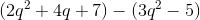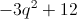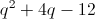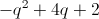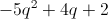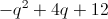Explanation:This is not a FOIL problem, as we are adding rather than multiplying the terms in parentheses.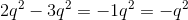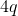has no like terms.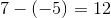Combine these terms into one expression to find the answer: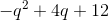### All SAT Math Resources